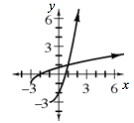### Home > INT3 > Chapter 8 > Lesson 8.3.1 > Problem8-96

8-96.

What is the inverse of $g\left(x\right) = \left(x + 1\right)^{2} – 3$ for $x\ge-1$? Sketch graphs of $g\left(x\right)$ and its inverse on the same set of axes, and then state the domain and range of the inverse function.

Let $g\left(x\right) = y$. Then interchange $x$ and $y$.
$x = \left(y + 1\right)^{2} − 3$

Add $3$ to both sides of the equation.
$x + 3 = \left(y + 1\right)^{2}$

Take the square root of both sides.

$y + 1 = \sqrt{x + 3}$

$g^{-1}(x) = \sqrt{x + 3} - 1$

D: $x\ge−3$
R: $y\ge−1$